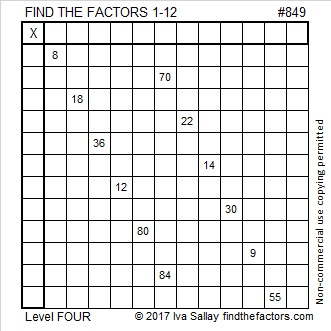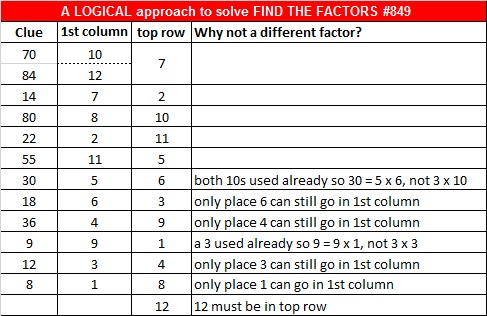# 849 and Level 4Print the puzzles or type the solution on this excel file: 12 factors 843-852

8 + 4 + 9 = 21, a number divisible by 3 so odd number 849 can be evenly divided by 3, AND 849 can be written as the sum of three consecutive numbers and six consecutive numbers.

• 282 + 283 + 284 = 849.
• 139 + 140 + 141 + 142 + 143 + 144 = 849

849 can also be written as the sum of three odd numbers: 281 + 283 + 285 = 849.

849 can be written as the difference of two squares two different ways:

• 143² – 140² = 849
• 425² – 424² = 849

True, similar things can be written about every other number that is divisible by 3, but they are still fun facts about the number 849.

• 849 is a composite number.
• Prime factorization: 849 = 3 × 283
• The exponents in the prime factorization are 1 and 1. Adding one to each and multiplying we get (1 + 1)(1 + 1) = 2 × 2 = 4. Therefore 849 has exactly 4 factors.
• Factors of 849: 1, 3, 283, 849
• Factor pairs: 849 = 1 × 849 or 3 × 283
• 849 has no square factors that allow its square root to be simplified. √849 ≈ 29.1376This site uses Akismet to reduce spam. Learn how your comment data is processed.